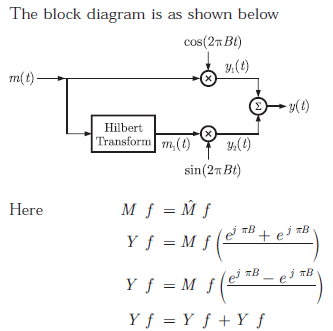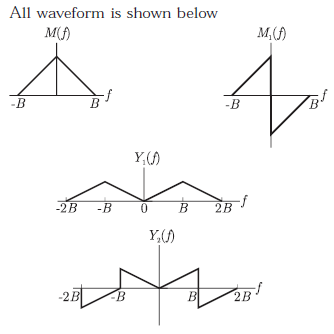# Communication Engineering - Online Test

Q1. A dual trace oscilloscope is set to operate in the Alternate mode. The control input of the multiplexer used in the y-circuit is fed with a signal having a frequency equal to
Explaination / Solution:
No Explaination.

Q2. If R(τ) is the auto correlation function of a real, wide-sense stationary random process, then which of the following is NOT true
Explaination / Solution:
No Explaination.

Q3. A plane wave of wavelength λ is traveling in a direction making an angle 300 with positive x − axis and 900 with positive y − axis. The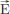field of the plane wave can be represented as (E is constant)
Explaination / Solution: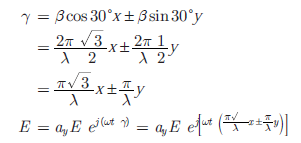Q4. The fourier series expansionof the periodic signal shown below will contain the following nonzero terms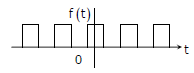Explaination / Solution:

⇒ it satisfies the half wave symmetry, so that it contains only odd harmonics.
⇒ It satisfies the even symmetry. So bn = 0

Q5. If S(f) is the power spectral density of a real, wide-sense stationary random process, then which of the following is ALWAYS true?
Explaination / Solution:
No Explaination.

Q6.
The steady state error of a unity feedback linear system for a unit step input is 0.1. The steady state error of the same system, for a pulse input r(t) having a magnitude of 10 and a duration of one second, as shown in the figure isExplaination / Solution:Q7. In delta modulation, the slope overload distortion can be reduced by
Explaination / Solution:
No Explaination.

Q8. Given two continuous time signals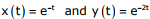which exist for t > 0, the convolution z(t) = x(t)* y(t) is
Explaination / Solution:Q9. The raised cosine pulse p(t) is used for zero ISI in digital communications. The expression for p(t) with unity roll-off factor is given byThe value of p(t) at t = 1/W is
Explaination / Solution: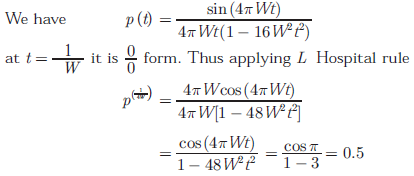Q10.
In the following scheme, if the spectrum M(f) of m(t) is as shown, then the spectrum Y(f) of y(t) will be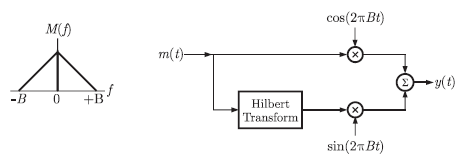Explaination / Solution: